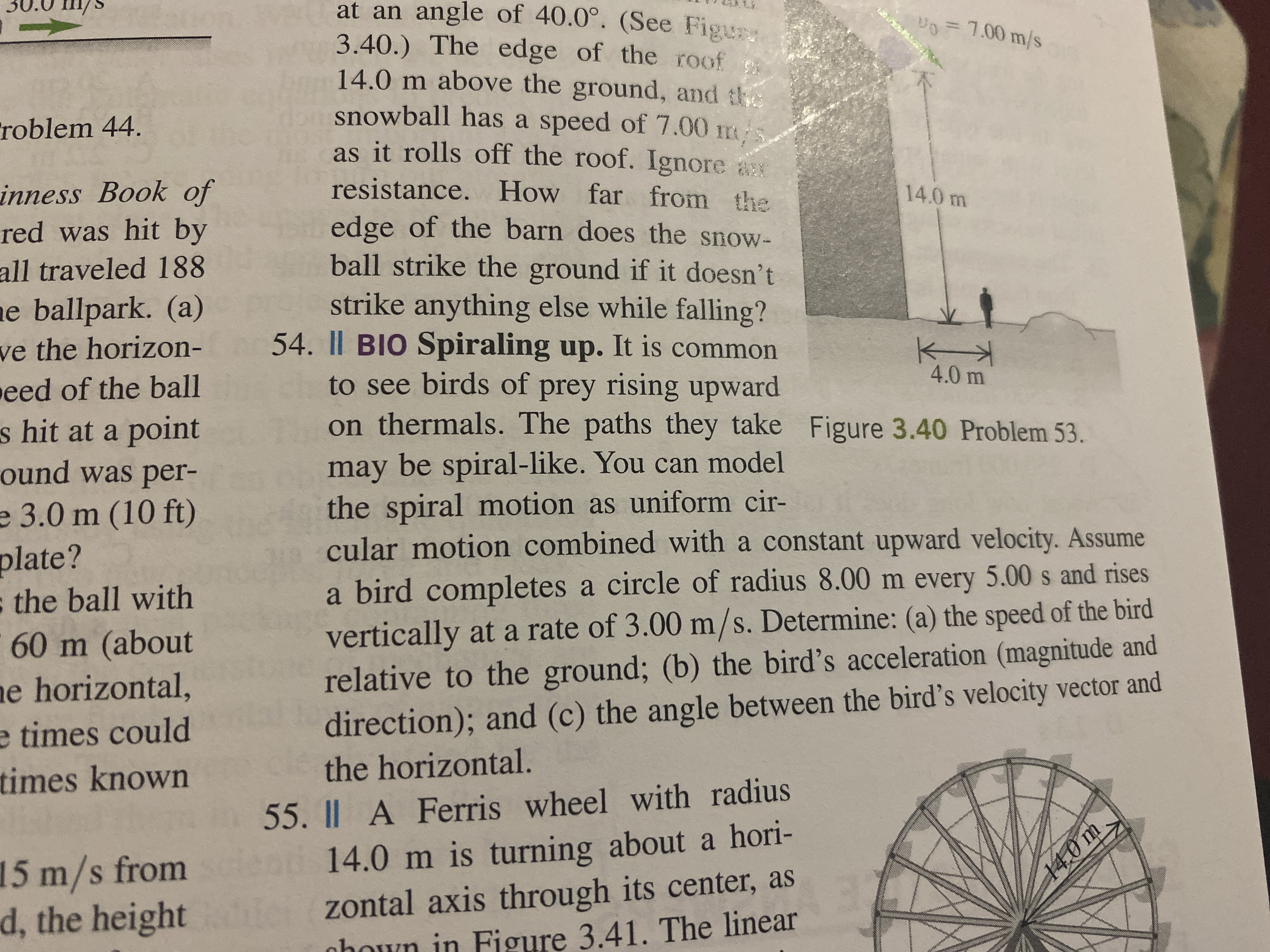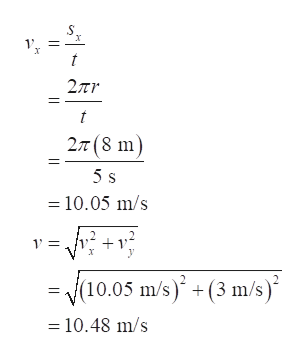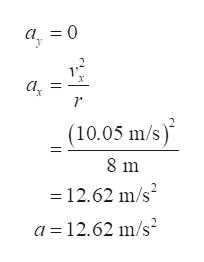# 30.0 /sat an angle of 40.0°. (See Figury3.40.) The edge of the Ioof14.0 m above the ground, and thesnowball has a speed of 7.00 mas it rolls off the roof. Ignore aV0 7.00 m/sroblem 44.resistance. How far from theinness Book ofred was hit by14.0 medge of the barn does the snow-ball strike the ground if it doesn'tstrike anything else while falling?54. Il BIO Spiraling up. It is commonto see birds of prey rising upwardon thermals. The paths they take Figure 3.40 Problem 53.all traveled 188ae ballpark. (a)ve the horizon-eed of the ball4.0 ms hit at a pointound was per-may be spiral-like. You can modelthe spiral motion as uniform cir-cular motion combined with a constant upward velocity. Assumee 3.0 m (10 ft)plate?the ball witha bird completes a circle of radius 8.00 m every 5.00 s and risesvertically at a rate of 3.00 m/s. Determine: (a) the speed of the birdrelative to the ground; (b) the bird's acceleration (magnitude anddirection); and (c) the angle between the bird's velocity vector and60 m (aboute horizontal,e times couldtimes knownthe horizontal.55. Il A Ferris wheel with radiushori-15 m/s fromd, the heightad14.0 m is turning aboutzontal axis through its center, asFigure 3.41. The linear14.0 m

Question
45 views

Question number 54.help_outlineImage Transcriptionclose30.0 /s at an angle of 40.0°. (See Figury 3.40.) The edge of the Ioof 14.0 m above the ground, and the snowball has a speed of 7.00 m as it rolls off the roof. Ignore a V0 7.00 m/s roblem 44. resistance. How far from the inness Book of red was hit by 14.0 m edge of the barn does the snow- ball strike the ground if it doesn't strike anything else while falling? 54. Il BIO Spiraling up. It is common to see birds of prey rising upward on thermals. The paths they take Figure 3.40 Problem 53. all traveled 188 ae ballpark. (a) ve the horizon- eed of the ball 4.0 m s hit at a point ound was per- may be spiral-like. You can model the spiral motion as uniform cir- cular motion combined with a constant upward velocity. Assume e 3.0 m (10 ft) plate? the ball with a bird completes a circle of radius 8.00 m every 5.00 s and rises vertically at a rate of 3.00 m/s. Determine: (a) the speed of the bird relative to the ground; (b) the bird's acceleration (magnitude and direction); and (c) the angle between the bird's velocity vector and 60 m (about e horizontal, e times could times known the horizontal. 55. Il A Ferris wheel with radius hori- 15 m/s from d, the height ad14.0 m is turning about zontal axis through its center, as Figure 3.41. The linear 14.0 m fullscreen
check_circle

Step 1

Let v be the velocity of the bird. Let vx  be the velocity in x-direction, and vy be the velocity in the vertical direction, and sx be the distance travelled by the bird in the horizontal direction.

Write the given values in the question.

Step 2

Write the expression for the horizontal and vertical components of the velocity and substitute known values to find the relative velocity of bird with respect to the ground which is its magnitude.help_outlineImage Transcriptionclose1 t 2πη 27t(8 m 5 s 10.05 m/s 2 1 = V10.05 m/s+(3 m/s) 10.48 m/s fullscreen
Step 3

As the bird is moving with constant velocity along the upward direction as mentioned in the question. Acceleration in vertical direction is zero. The only acceleration will be ...help_outlineImage Transcriptioncloseа, 3 0 х а, (10.05 m/s) 2 8 m 12.62 m/s2 а %3D12.62 m/s? fullscreen

### Want to see the full answer?

See Solution

#### Want to see this answer and more?

Solutions are written by subject experts who are available 24/7. Questions are typically answered within 1 hour.*

See Solution
*Response times may vary by subject and question.
Tagged in

### Angular Motion